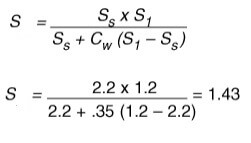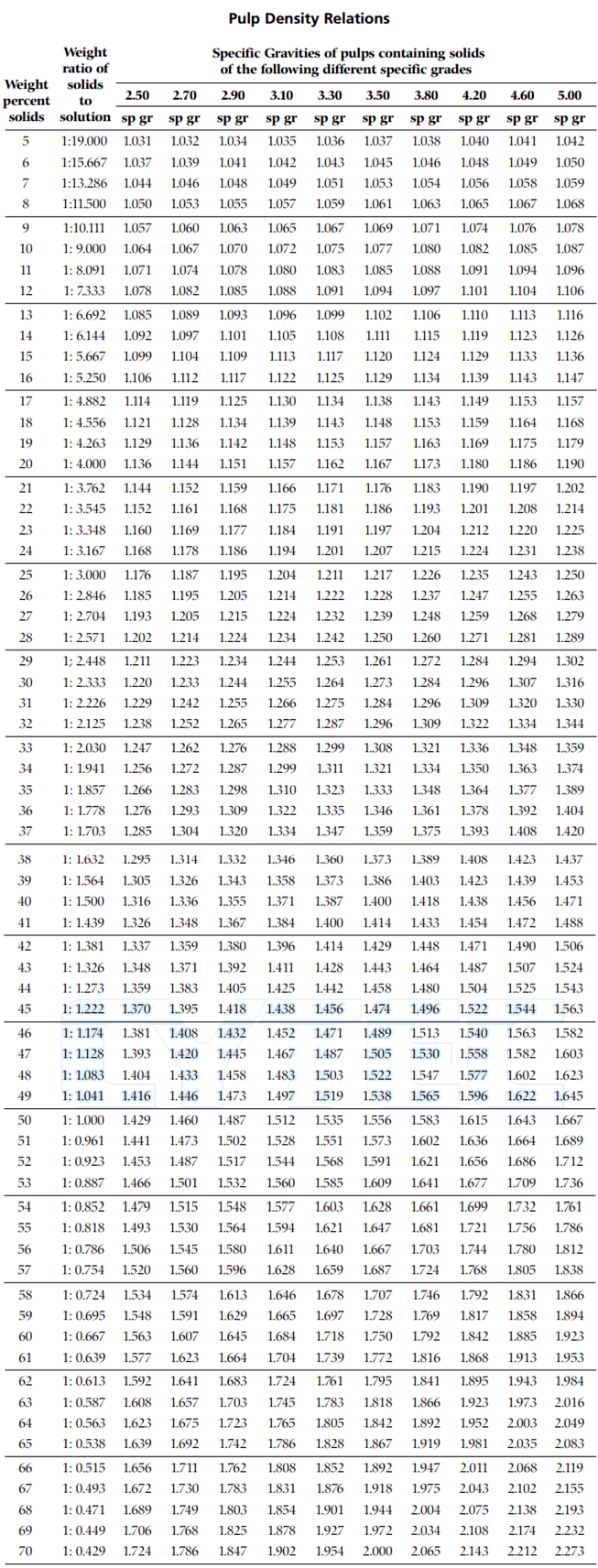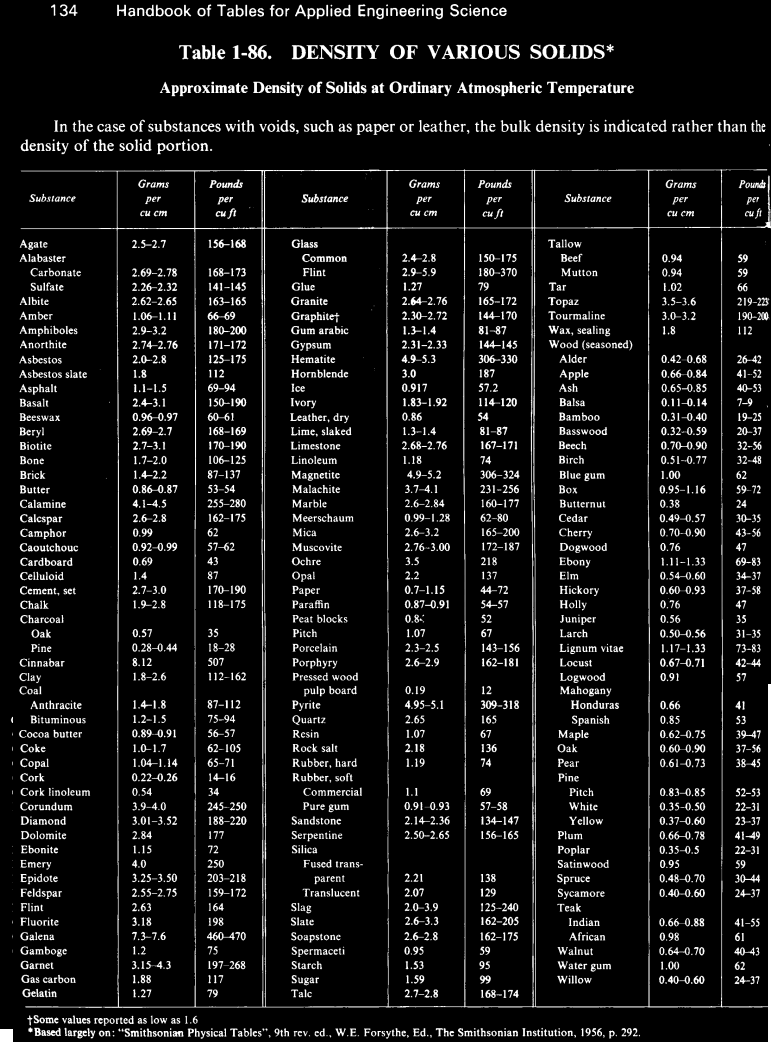# Percent Solids to Specific Gravity Conversion of Slurry

Here is the formula for specific gravity of a slurry, S is:

S = specific gravity of slurry
Si= specific gravity of liquid phase
Ss= specific gravity of solids phase
Cw = concentration of solids by weight
Cv= concentration of solids by volume
EXAMPLE: if the liquid has a specific gravity of 1.2 and the concentration of solids by weight is 35% with
the solids having a specific gravity of 2.2, then:You will also find this Nomograph useful.1 MINERAL PROCESSING FORMULASW/S Ratio.—The mixture of ore and water in which the process of concentrating the valuable minerals is performed is always termed a PULP. Its constitution can be expressed by stating that it contains a certain percentage of solid particles or “ solids ”, but just as common a method of describing the proportion of water to ore is by expressing it as a Water/Solids or W/S ratio, the amount of solids always being taken as unity. Thus a pulp containing 25% of solids has a W/S ratio of 3/1.